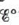Chapter 18, Problem 53E

Chapter
Section
Textbook Problem

# Estimatefor the half-reaction 2 H 2 O  +   2 e − →  H 2 +   2 OH − given the following values of Δ G f o : H 2 O ( l )   =   − 237  kJ/mol H 2 ( g )   =   0.0 OH − ( a q ) =   − 157  kJ/mol e − =   0.0 Compare this value ofwith the value ofgiven in Table 17-1.

Interpretation Introduction

Interpretation:

A half-reaction is given. The comparison of the value of E° calculated from the given data is to be stated.

Concept introduction:

The species with higher reduction potential undergoes reduction at the cathode while the species with lower reduction potential undergoes oxidation at the anode. The change in Gibbs free energy is the useful amount work that can be done and negative value of ΔG° shows that the reaction is spontaneous in nature.

To determine: The value of E° for the given half-reaction and to compare it with the value given in Table 171 .

Explanation

The given half-cell reaction is,

2H2O(l)+2eH2(g)+2OH(aq)

The value of ΔG° is calculated using the formula,

ΔG°=npΔG°f(products)nrΔG°f(reactants)

Where,

• ΔG° is the Gibbs free energy change for the entire cell.
• ΔG°f(products) is the Gibbs free energy change for the products.
• ΔG°f(reactants) is the Gibbs free energy change for the reactants.
• np is the total number of moles of the products.
• nr is the total number of moles of the reactants.

Substitute the given values in the above equation,

ΔG°=ΔG°f(H2(g))+2ΔG°f(OH(aq))2ΔG°f(H2O(l))2ΔG°f(e)=[1mol(0.0kJmol)+2mol(157kJmol)2mol(237kJmol)2mol(0

### Still sussing out bartleby?

Check out a sample textbook solution.

See a sample solution

#### The Solution to Your Study Problems

Bartleby provides explanations to thousands of textbook problems written by our experts, many with advanced degrees!

Get Started